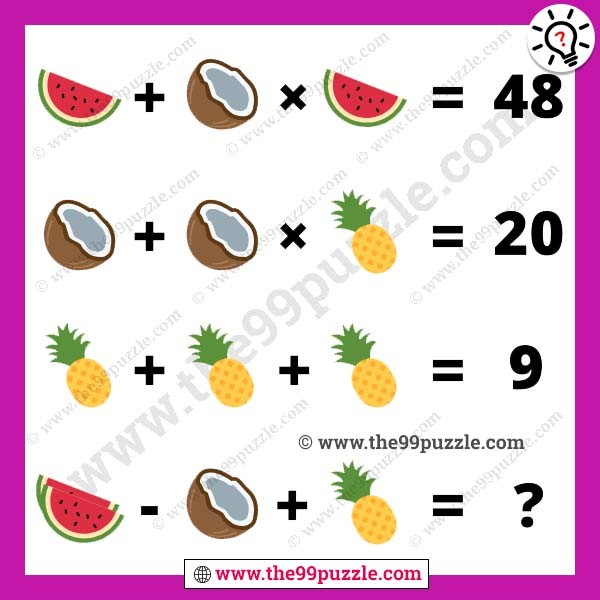# Fun and tricky picture math puzzle – Puzz231

Fun and tricky picture math puzzle for logical students, who find this type of puzzle. Picture math equations are the most interesting brain game. When you try to solve these puzzles, you will get more fun and learn to solve them quickly. Puzzles make you smarter when you solve very quickly. In this picture puzzle, you will see watermelon, coconut, and pineapple in the math equation. You have to find each value and solve the last picture equation.###### Explanation:

Watermelon + Coconut × Watermelon = 48

Coconut + Coconut × Pineapple = 20

Pineapple + Pineapple + Pineapple = 9

(2×Watermelon) – Coconut + Pineapple = ?

Watermelon = 8

Coconut = 5

Pineapple = 3

8 + 5 × 8 = 48

5 + 5 × 3 = 20

3 + 3 + 3 = 9

(2×8) – 5 + 3 = 14